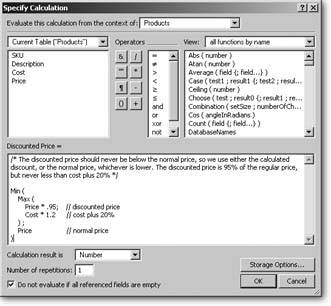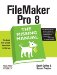# Going Beyond Basic Calculations

In the last chapter you created two very simple calculation fields using numbers: Extended Price and Invoice Total. But those calculations were very simple. Now, you build on those basic concepts to see how you can make a set of calculations that fit an upcoming sales promotion.

10.2.1. Preparing the Data

You've decided to start reselling personal security products to your customers. To help you keep track of things, you need to add a Products table to your database. The table should have these fields:

• A text field called SKU
• A text field called Description
• A number field called Cost
• A number field called Price

Note: See Section 7.3 for advice on creating tables.

Once you've defined this new table, FileMaker automatically creates a layoutProducts. Switch to that layout now and create a few product records. To help drive sales to your larger clients, you want to implement a volume discount schemethey should get a 5 percent discount if they buy enough. But some of the products you sell don't have enough mark-up to justify these discounts. You want to be sure the discount never reduces your mark-up below 20 percent.

First, you add a line to your marketing materials: "Volume discounts not available for all products." You can make this line as small as humanly possible and hide it way down in the corner. Next, you need to fix your database so it tells you the discount price for each product.

10.2.2. Building the Calculation Fields

To implement this discount scheme, take what you need to know and translate it into calculation terms. You need three fields to carry out your sales promotion logic.

• First, calculate 95 percent of the price (a 5 percent discount):

```	Price Each * .95
```
• Second, you also know the cost (in the Cost field) and you can figure out the lowest price by adding 20 percent to this cost:

```	Cost * 1.2
```
• Finally, the discounted price is either the calculated discount price, or the cost + 20 percent price, whichever is greater. Put another way, you want the maximum of these two values:

```	Max ( Price Each * .95 ; Cost * 1.2 )
```

Using the Max function, the previous calculation results in either the discounted price, or the minimum price, whichever is greater (see the box on Section 10.2.3). That result's almost perfect. But suppose you have a product whose normal price is less than 20 percent above cost (hey, it's a competitive market). If you use the Max calculation as it is now, the new discounted price is more than the normal price. You need to go back and add to your calculation so that it takes the regular price into account, and uses that price if it's lower than the calculated discount. Read on to learn how to think through this calculation quandary.

 UP TO SPEED The Max and Min Functions Many times, you need to know either the highest or lowest value in a series. The Max function and its twin sister, Min, fulfill these needs and you find them in the function list's Aggregate functions category. Like all the aggregate functions, they expect at least one parameter, and are glad to get more. Every parameter should be a number. Your parameters can be: Constant data Fields within a record Repeating fields Related fields The Max function looks at every number referenced and returns whichever is largest. Min, on the other hand, returns the smallest value. For example, look at this calculation: ``` Max ( 10 ; 3 ; 72 ; 19 ; 1 ) ```   Its result is 72, since that's the largest of the parameters. If you had a repeating field called Distances that held the distances from your office to each Krispy Kreme store, you could use this calculation to find the closest sugar fix: ``` Min ( Distances ) ```   The same is true for related fields too. This calculation finds the most expensive line item: ``` Max ( Line Items::Price ) ```   For another example of an aggregate function, Sum, see Section 9.1.1.

10.2.3. Constructing the Calculation

When calculations start to get complicated like this discount price example, imagine that you have a field that contains the value you already want. You can use this pretend field in your new calculation, and then, when you're all finished, put the old calculation in place of the pretend field. In this case, just pretend you have a field called Calculated Discount that holds the discount price. With that imaginary field in mind, you can fix your problem with this calculation:

```	Min ( Calculated Discount ; Price Each )
```

The result of this calculation is either the calculated discount or the regular price, if it's lower. Now, just put the old calculation in place of the words "Calculated Discount" (since the old calculation results in the calculated discount):

```	Min ( Max ( Price Each * .95 ; Cost * 1.2 ) ; Price Each )
```

The entire Max function, complete with its two parameters, is now the Min function's first parameter. You might think it looks a little confusing at first, but with practice you become accustomed to looking at functions-inside-functions like this.

If it helps, you can add white space and comments to clarify the calculation, as Figure 10-2 shows.Figure 10-2. You may find it hard to write nested calculations, and hard to read them if you need to come back later and tweak them. In this case, though, a savvy person formatted the calculation with copious white space and plenty of comments. Chances are you'll understand this version better than the one shown on Section 10.2.3.

To use the calculation, just create a new calculation field (call it Discounted Price) with the calculation above. It automatically adjusts and shows you an appropriate discounted price.

If you want to test a complicated calculation, spot-check a few records where you know the result. Sometimes the math is so complex that you just have to work it out on paper and enter dummy records to check the calculation. Usually, if a calculation isn't working, you can figure out how to fix it when you compare your math to the value in the field.FileMaker Pro 8: The Missing Manual
ISBN: 0596005792
EAN: 2147483647
Year: 2004
Pages: 176